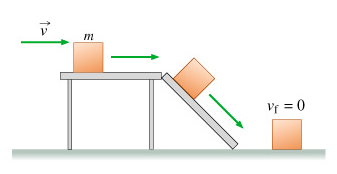# Problem:  A block of mass m slides at a speed v along a horizontal, smooth table. It next slides down a smooth ramp, descending a height h, and then slides along a horizontal rough floor, stopping eventually. Assume that the block slides slowly enough so that it does not lose contact with the supporting surfaces (table, ramp, or floor).You will analyze the motion of the block at different moments using the law of conservation of energy.Part BSuppose the potential energy of the block at the table is given by mgh/3. This implies that the chosen zero level of potential energy is __________.a. a distance h/3 above the floora. a distance h/3 below the floora. a distance 2h/3 above the floora. a distance 2h/3 below the floora. on the floorPart CIf the zero level is a distance 2h/3 above the floor, what is the potential energy U of the block on the floor? Express your answer in terms of some or all the variables m, v, and hand any appropriate constants.Part DConsidering that the potential energy of the block at the table is mgh/3 and that on the floor is ?2mgh/3, what is the change in potential energy? U of the block if it is moved from the table to the floor? Express your answer in terms of some or all the variables m, v, and hand any appropriate constants.

###### FREE Expert Solution

Part B

From the law of conservation of energy:

mgh = mgh/3 + mgh'###### Problem Details

A block of mass m slides at a speed v along a horizontal, smooth table. It next slides down a smooth ramp, descending a height h, and then slides along a horizontal rough floor, stopping eventually. Assume that the block slides slowly enough so that it does not lose contact with the supporting surfaces (table, ramp, or floor).

You will analyze the motion of the block at different moments using the law of conservation of energy.Part B

Suppose the potential energy of the block at the table is given by mgh/3. This implies that the chosen zero level of potential energy is __________.

a. a distance h/3 above the floor

a. a distance h/3 below the floor

a. a distance 2h/3 above the floor

a. a distance 2h/3 below the floor

a. on the floor

Part C

If the zero level is a distance 2h/3 above the floor, what is the potential energy U of the block on the floor? Express your answer in terms of some or all the variables m, v, and hand any appropriate constants.

Part D

Considering that the potential energy of the block at the table is mgh/3 and that on the floor is ?2mgh/3, what is the change in potential energy? U of the block if it is moved from the table to the floor? Express your answer in terms of some or all the variables m, v, and hand any appropriate constants.